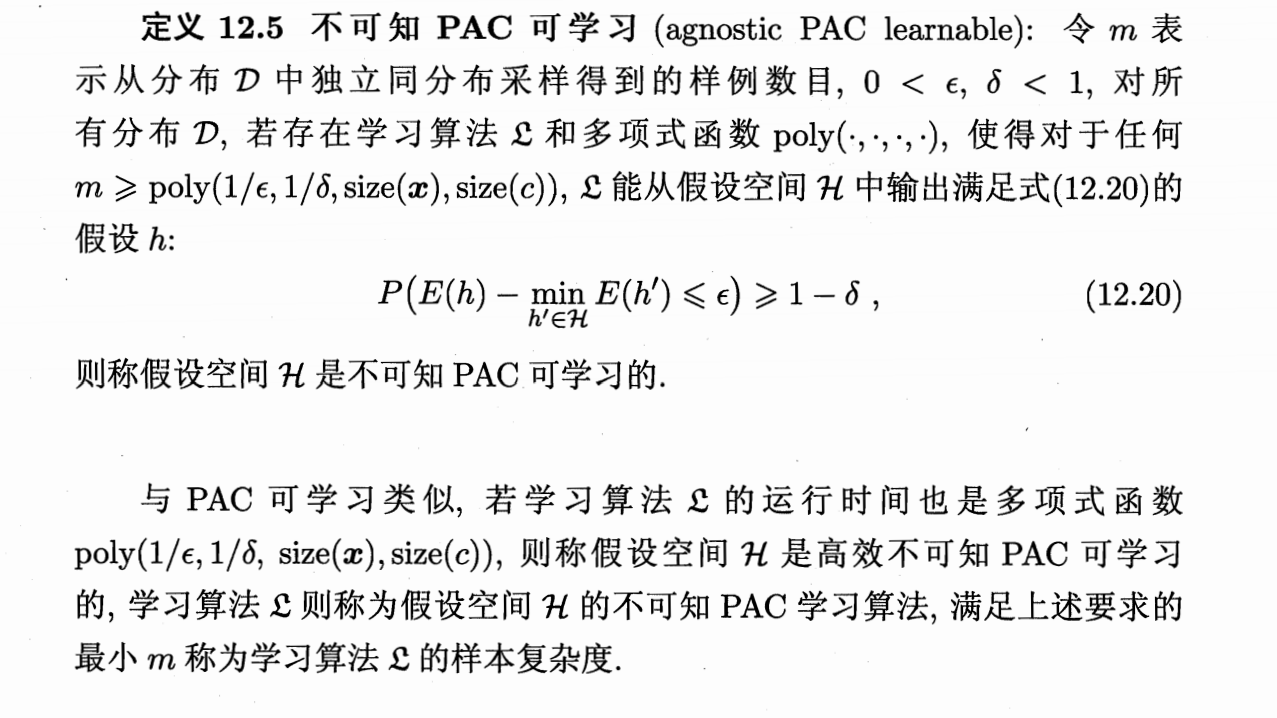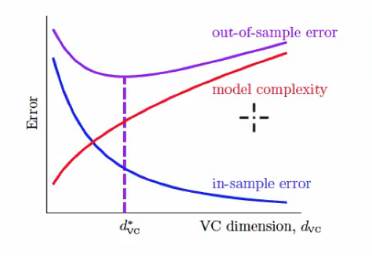## 基础知识

Coursera上有一门很热门的台湾课程<机器学习基石>, 其上半部分都是在讲这部分内容, 足见其重要性.

$h$为$\mathcal{X \rightarrow Y}$的映射, 泛化误差为:

$h$在$D$上的经验误差为:

1. Jensen 不等式：
1. Hoeffding不等式, 对$m$个随机变量$x_1, …, x_m$， $0\le x_i \le 1$, 则对任意$\epsilon \gt 0$
1. McDiarmid不等式，对任意$1\le i\le m$，函数$f$满足：

## PAC学习

PAC理论(概率近似正确 Probably Approximately Correct)是学习理论中最基本的理论.

• $c$表示概念(concept), 表示样本空间到标记空间的映射, 若有对任何样例$(x,y)$有$C(x)=y$,则称$c$为目标概念, 所有目标概念的集合称为概念类$\mathcal{C}$
• $\mathcal{L}$为学习算法, 其能表示的所有可能概念的集合称为假设空间, 用$\mathcal{H}$表示. 由于$\mathcal{H},\mathcal{C}$通常不同, 所以对于$h\in \mathcal{H}$我们不确定其是否为目标概念,则称之为假设.
• 若假设空间存在至少一个目标概念, 则称该问题对算法$\mathcal{L}$可分,一致, 否则即为不可分,不一致.

### 不可分情况## VC维

1. 增长函数
对于假设$h$, 我们用它来对样本集赋予标记, 可以得到 随着$m$ 增大, 假设空间中所有假设对D中样本所能赋予的标记数的可能结果也会增加. 我们定义增长函数为: 也就是假设空间对样本集所能赋予标记的最大可能结果数. 增长函数能够体现假设空间的表达能力和复杂度, 有定理如下:
2. 打散
如果假设空间能够实现数据集上所有”对分”(我们称假设对数据集中数据赋予标记的每种可能结果称作对分), 对于二分类问题即为增长函数为$2^m$, 则称示例集能被假设空间打散.## 稳定性

• $D^{\backslash i}$表示移除第$i$个样本后的集合
• $D^i$表示替换第$i$个样本后的集合

• 泛化损失

• 经验损失

• 留一(leave-one-out)损失